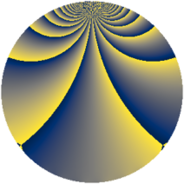# Properties

 Label 3630.2.bkLevel $3630$ Weight $2$ Character orbit 3630.bk Rep. character $\chi_{3630}(31,\cdot)$ Character field $\Q(\zeta_{55})$ Dimension $3520$ Sturm bound $1584$

# Related objects

## Defining parameters

 Level: $$N$$ $$=$$ $$3630 = 2 \cdot 3 \cdot 5 \cdot 11^{2}$$ Weight: $$k$$ $$=$$ $$2$$ Character orbit: $$[\chi]$$ $$=$$ 3630.bk (of order $$55$$ and degree $$40$$) Character conductor: $$\operatorname{cond}(\chi)$$ $$=$$ $$121$$ Character field: $$\Q(\zeta_{55})$$ Sturm bound: $$1584$$

## Dimensions

The following table gives the dimensions of various subspaces of $$M_{2}(3630, [\chi])$$.

Total New Old
Modular forms 32000 3520 28480
Cusp forms 31360 3520 27840
Eisenstein series 640 0 640

## Trace form

 $$3520q + 88q^{4} - 880q^{9} + O(q^{10})$$ $$3520q + 88q^{4} - 880q^{9} - 16q^{10} - 28q^{11} - 104q^{13} - 84q^{14} + 88q^{16} - 8q^{17} + 8q^{19} + 8q^{22} + 16q^{23} + 88q^{25} + 8q^{26} - 24q^{29} + 4q^{30} - 88q^{31} + 8q^{33} + 8q^{34} + 8q^{35} + 88q^{36} - 24q^{37} + 16q^{38} + 8q^{39} - 40q^{40} + 4q^{41} - 16q^{43} + 12q^{44} - 4q^{46} - 24q^{47} + 20q^{49} - 8q^{51} + 24q^{52} - 56q^{53} - 8q^{55} + 24q^{56} + 8q^{57} - 72q^{58} + 24q^{59} - 16q^{61} - 24q^{62} + 88q^{64} + 8q^{65} - 8q^{66} - 72q^{67} - 8q^{68} + 16q^{69} + 48q^{71} - 152q^{73} - 40q^{74} - 36q^{76} - 80q^{77} - 228q^{79} - 880q^{81} - 120q^{82} - 8q^{83} + 8q^{85} + 16q^{86} + 16q^{87} - 32q^{88} - 72q^{89} + 4q^{90} - 204q^{91} + 16q^{92} + 16q^{93} - 188q^{94} - 240q^{97} + 32q^{98} + 12q^{99} + O(q^{100})$$

## Decomposition of $$S_{2}^{\mathrm{new}}(3630, [\chi])$$ into newform subspaces

The newforms in this space have not yet been added to the LMFDB.

## Decomposition of $$S_{2}^{\mathrm{old}}(3630, [\chi])$$ into lower level spaces

$$S_{2}^{\mathrm{old}}(3630, [\chi]) \cong$$ $$S_{2}^{\mathrm{new}}(121, [\chi])$$$$^{\oplus 8}$$$$\oplus$$$$S_{2}^{\mathrm{new}}(242, [\chi])$$$$^{\oplus 4}$$$$\oplus$$$$S_{2}^{\mathrm{new}}(363, [\chi])$$$$^{\oplus 4}$$$$\oplus$$$$S_{2}^{\mathrm{new}}(605, [\chi])$$$$^{\oplus 4}$$$$\oplus$$$$S_{2}^{\mathrm{new}}(726, [\chi])$$$$^{\oplus 2}$$$$\oplus$$$$S_{2}^{\mathrm{new}}(1210, [\chi])$$$$^{\oplus 2}$$$$\oplus$$$$S_{2}^{\mathrm{new}}(1815, [\chi])$$$$^{\oplus 2}$$